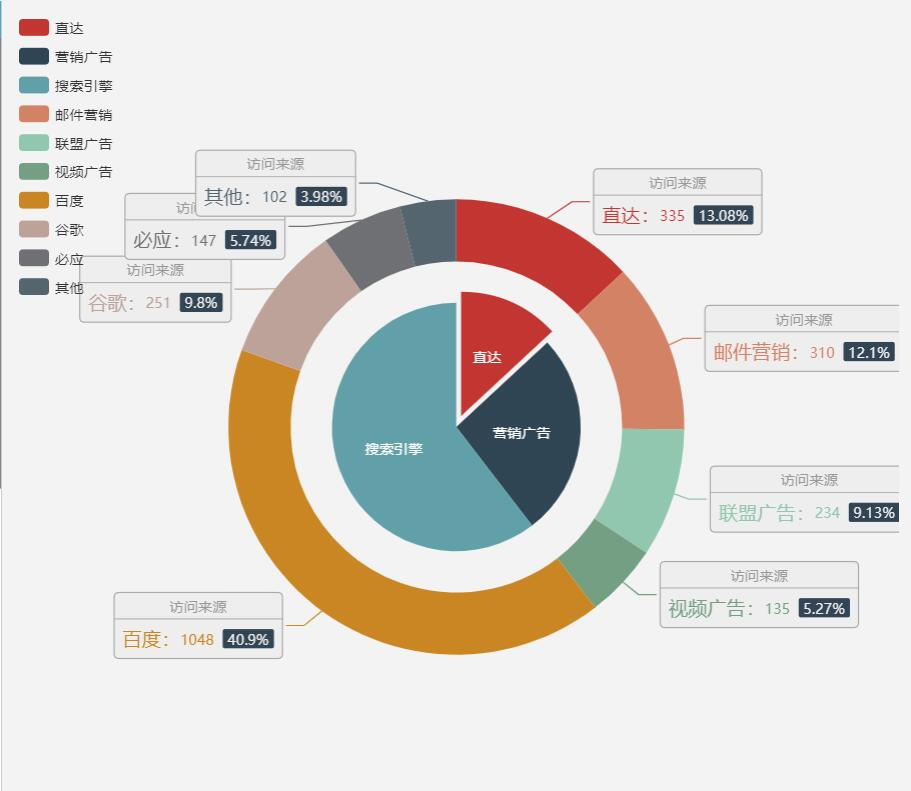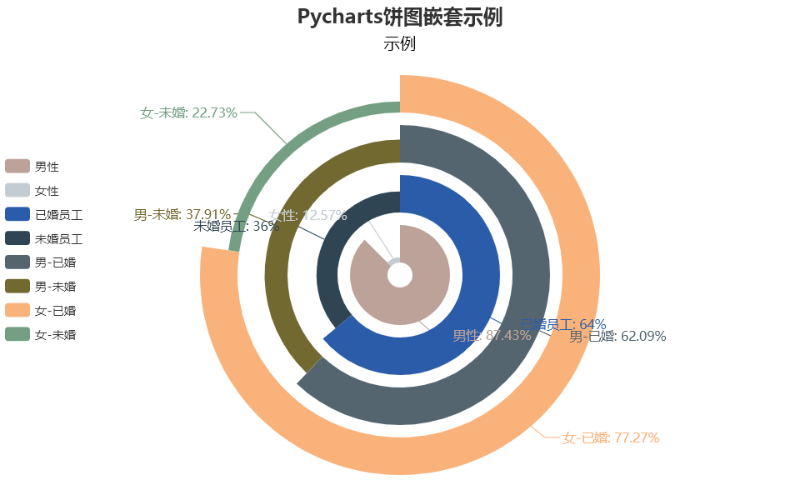## Python+Echarts=Pyecharts 图表运用：嵌套饼图的自行创建、运用示例（含完整可运行源码）

2021/1/9 2:17:02 文章标签: 复合条饼图

### version 1.1.0 - 2019.5.14

Add

• issue#1052，issue#1065 新增 BMap 图形种类
• issue#1078，issue#396 新增 Sunburst 图形种类
• issue#1112 Page 类新增 render_embed 方法
• ···

也就是说，Sunburst这个图表类型是在1.1.0这个版本中才加入的，0.5.11不能使用。无奈，因为也就是性别和婚姻的情况，如果用柱状图的stack会显得比较单调，最后是采用了：饼图嵌套

Echarts“饼图嵌套示例”：https://echarts.baidu.com/examples/editor.html?c=pie-nest``````from pyecharts import Pie
a1 = ["男性", "女性"]
v1 = [459,66]

a11=["已婚员工","未婚员工"]
v11=[336,189]

a21=["男-已婚","男-未婚"]
v21=[285,174]

a22=["女-已婚","女-未婚"]
v22=[51,15]
pie= Pie('Pycharts饼图嵌套示例','示例',title_pos='center',title_text_size=20,subtitle_color= '#000',subtitle_text_size=16,height=500)

pie.add('', a1, v1, center=[50,55],radius=[5,20],is_random=True,
is_label_show=True,label_text_size=13,label_pos='bottom',
legend_pos='left',is_more_utils=True,rosetype='radius'
,label_emphasis_pos='top',label_emphasis_textcolor='',label_emphasis_textsize=30
)

pie.add('',a11, v11, center=[50,55],radius=[25,40] ,
is_label_show=True,label_text_size=13,label_pos='top',
legend_pos='left',rosetype='radius',label_emphasis_pos='top'
,label_emphasis_textcolor='',label_emphasis_textsize=30
)

pie.add('',a21, v21, center=[50,55],radius=[45,60] ,label_pos='right',
is_label_show=True,label_text_size=13,legend_pos='left',rosetype='radius'
,label_emphasis_pos='top',label_emphasis_textcolor='',label_emphasis_textsize=30)

pie.add('',a22, v22,center=[50,55], radius=[65,80] ,label_pos='left',
is_label_show=True,label_text_size=13,
legend_pos='left',legend_top='center',rosetype='radius',legend_orient="vertical"
,label_emphasis_pos='top',label_emphasis_textcolor='',label_emphasis_textsize=30)

pie.render()``````

暂无相关的数据...